Next: Data Acquisition and Process Up: Digital Circuits Previous: Divide-by-N Counters

# Problems

1. Using only two-input NOR gates, show how AND, OR and NAND gates can be made.
2. The binary addition of two 2-bit numbers (with carry bits) looks like the following: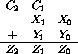1. Write a truth table expressing the outputs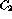and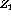as a function of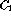,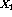and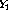.
2. Write an algebraic statement in Boolean algebra describing this truth table.
3. Implement this statement using standard (AND, OR, EX-OR and inverter gates).
3. If the 3-bit binary number A B C represents the digits 0 to 7:
1. Make a truth table for A, B, C and Q, where Q is true only when an odd number of bits are true in the number.
2. Write a statement in Boolean algebra for Q.
3. Convert this equation to one that can be mechanized using only two XOR gates. Draw the resulting circuit.
4. You need to provide a logic signal to control an experiment. The experiment is controlled by the four signals A, B, C and D, which make up the data word A B C D. The control line Q should be set high only if this data word takes on the values 1, 3, 5, 7, 11 or 13.
1. Write a truth table for this function.
2. Using boolean algebra, write an expression indicating when Q is true. Your statement should include one logical statement for each of the six true conditions, each separated by the OR function. Therefore this statement should utilize a number of AND/NAND functions, and five OR statements.
3. Simplify this statement so that it can be implemented using two two-input AND gates, one OR gate, one exclusive OR gate and one logical inverter. The expression has the form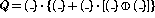, where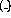stands for any of the four input signals or their logical inverses.
4. Implement this simplified function using logic gates.Next: Data Acquisition and Process Up: Digital Circuits Previous: Divide-by-N Counters

Doug Gingrich
Tue Jul 13 16:55:15 EDT 1999